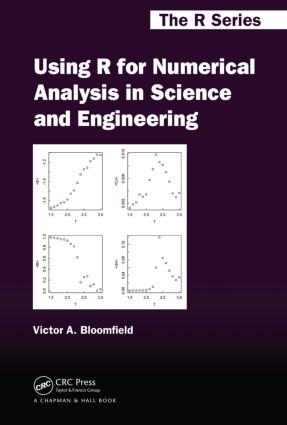# Using R for Numerical Analysis in Science and Engineering

## 1st Edition

Chapman and Hall/CRC

360 pages | 133 B/W Illus.

##### Purchasing Options:\$ = USD
Hardback: 9781439884485
pub: 2014-04-24
SAVE ~\$21.00
\$105.00
\$84.00
x
eBook (VitalSource) : 9781315373799
pub: 2018-09-03
from \$49.48

FREE Standard Shipping!

### Description

Instead of presenting the standard theoretical treatments that underlie the various numerical methods used by scientists and engineers, Using R for Numerical Analysis in Science and Engineering shows how to use R and its add-on packages to obtain numerical solutions to the complex mathematical problems commonly faced by scientists and engineers. This practical guide to the capabilities of R demonstrates Monte Carlo, stochastic, deterministic, and other numerical methods through an abundance of worked examples and code, covering the solution of systems of linear algebraic equations and nonlinear equations as well as ordinary differential equations and partial differential equations. It not only shows how to use R’s powerful graphic tools to construct the types of plots most useful in scientific and engineering work, but also:

• Explains how to statistically analyze and fit data to linear and nonlinear models
• Explores numerical differentiation, integration, and optimization
• Describes how to find eigenvalues and eigenfunctions
• Discusses interpolation and curve fitting
• Considers the analysis of time series

Using R for Numerical Analysis in Science and Engineering provides a solid introduction to the most useful numerical methods for scientific and engineering data analysis using R.

### Reviews

"… the book is well organized, clearly written, and has a large amount of useful R code. It does a good job of answering the question of how to use R to perform numerical analyses of interest to scientists and engineers and, as such, can be recommended to the intended audience."

Journal of the Royal Statistical Society, Series A, 2015

"I would recommend it to those seeking to improve their programming efficiency. … the extensive coverage of optimization, ordinary differential equations, and partial differential equations combined with its exemplary demonstration of R coding through effective examples make this book a valuable resource for a wide audience. … a good reference for scientific and engineering researchers."

The American Statistician, February 2015

"… the book is well organized, clearly written, and has a large amount of useful R code. It does a good job answering the question of how to use R to perform numerical analyses of interest to scientists and engineers, and as such, can be recommended to the intended audience."

—Andrey Kostenko, Teaching Statistics

Introduction

Obtaining and Installing R

Learning R

Learning Numerical Methods

Finding Help

Augmenting R with Packages

Learning More about R

Calculating

Basic Operators and Functions

Complex Numbers

Numerical Display, Round-Off Error, and Rounding

Assigning Variables

Relational Operators

Vectors

Matrices

Time and Date Calculations

Graphing

Scatter Plots

Function Plots

Other Common Plots

Customizing Plots

Error Bars

Superimposing Vectors in a Plot

Modifying Axes

Adding Text and Math Expressions

Placing Several Plots in a Figure

Two- and Three-Dimensional Plots

The Plotrix Package

Animation

Programming and Functions

Conditional Execution: If and If Else

Loops

User-Defined Functions

Debugging

Built-in Mathematical Functions

Special Functions of Mathematical Physics

Polynomial Functions in Packages

Case Studies

Solving Systems Of Algebraic Equations

Finding the Zeroes of a Polynomial

Finding the Zeroes of a Function

Systems of Linear Equations: Matrix Solve

Matrix Inverse

Singular Matrix

Overdetermined Systems and Generalized Inverse

Sparse Matrices

Matrix Decomposition

Systems of Nonlinear Equations

Case Studies

Numerical Differentiation and Integration

Numerical Differentiation

Numerical Integration

Symbolic Manipulations in R

Case Studies

Optimization

One-Dimensional Optimization

Multi-Dimensional Optimization with Optim()

Other Optimization Packages

Optimization with Constraints

Global Optimization with Many Local Minima

Linear and Quadratic Programming

Mixed-Integer Linear Programming

Case Study

Ordinary Differential Equations

Euler Method

Improved Euler Method

deSolve Package

Matrix Exponential Solution for Sets of Linear ODEs

Events and Roots

Difference Equations

Delay Differential Equations

Differential Algebraic Equations

rootSolve for Steady State Solutions of Systems of ODEs

bvpSolve Package for Boundary Value ODE Problems

Stochastic Differential Equations: Gillespiessa Package

Case Studies

Partial Differential Equations

Diffusion Equation

Wave Equation

Laplace’s Equation

Solving PDEs with the Reactran Package

Examples with the Reactran Package

Case Studies

Analyzing Data

Getting Data into R

Data Frames

Summary Statistics for a Single Data Set

Statistical Comparison of Two Samples

Chi-Squared Test for Goodness of Fit

Correlation

Principal Component Analysis

Cluster Analysis

Case Studies

Fitting Models To Data

Fitting Data with Linear Models

Fitting Data with Nonlinear Models

Inverse Modeling of ODEs with the FME Package

Improving the Convergence of Series: Padé and Shanks

Interpolation

Time Series, Spectrum Analysis, and Signal Processing

Case Studies

### About the Author

Victor A. Bloomfield is currently emeritus professor at University of Minnesota, Minneapolis, USA. His research has encompassed more than four decades and a variety of topics, including enzyme kinetics, dynamic laser light scattering, bacteriophage assembly, DNA condensation, scanning tunneling microscopy, and single molecule stretching experiments on DNA. His theoretical work on biopolymer hydrodynamics and polyelectrolyte behavior has resulted in over 200 peer-reviewed journal publications. Using R for Numerical Analysis in Science and Engineering is an extension and broadening of his 2009 book, Computer Simulation and Data Analysis in Molecular Biology and Biophysics: An Introduction Using R, for general usage in science and engineering.

### Subject Categories

##### BISAC Subject Codes/Headings:
MAT000000
MATHEMATICS / General
MAT021000
MATHEMATICS / Number Systems
MAT029000
MATHEMATICS / Probability & Statistics / General